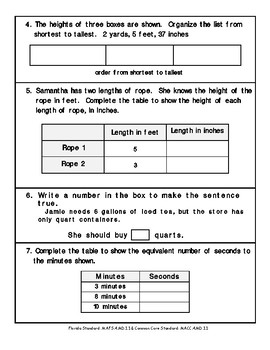# 4th Grade Math FSA Assessment for Measurement and Data {Bundle}Subject
Resource Type
File Type
PDF (1 MB|33 pages)
Standards
\$8.00
• Product Description
• Standards
This tests addresses standards MAFS.4.MD's and MACC.4.MD's covering Measurement and Data. A bundle of our seven tests with a \$1.00 savings!

Are you frustrated with not having summative assessments that are aligned to the new Florida Standards Assessments (FSA)? Well, look no further. Our assessments were created with the new Florida Standards Assessment in mind. Each standard includes 10 parallel type questions that are found on the Florida Item Specifications or Deconstructed Standards for Common Core. Our assessments will help prepare students for the tasks required on the new assessment, such as; multiple choice response, multi-select response, equation response, matching item response, and table response.
Recognize angle measure as additive. When an angle is decomposed into non-overlapping parts, the angle measure of the whole is the sum of the angle measures of the parts. Solve addition and subtraction problems to find unknown angles on a diagram in real world and mathematical problems, e.g., by using an equation with a symbol for the unknown angle measure.
Measure angles in whole-number degrees using a protractor. Sketch angles of specified measure.
An angle that turns through 𝘯 one-degree angles is said to have an angle measure of 𝘯 degrees.
An angle is measured with reference to a circle with its center at the common endpoint of the rays, by considering the fraction of the circular arc between the points where the two rays intersect the circle. An angle that turns through 1/360 of a circle is called a “one-degree angle,” and can be used to measure angles.
Recognize angles as geometric shapes that are formed wherever two rays share a common endpoint, and understand concepts of angle measurement:
Total Pages
33 pages